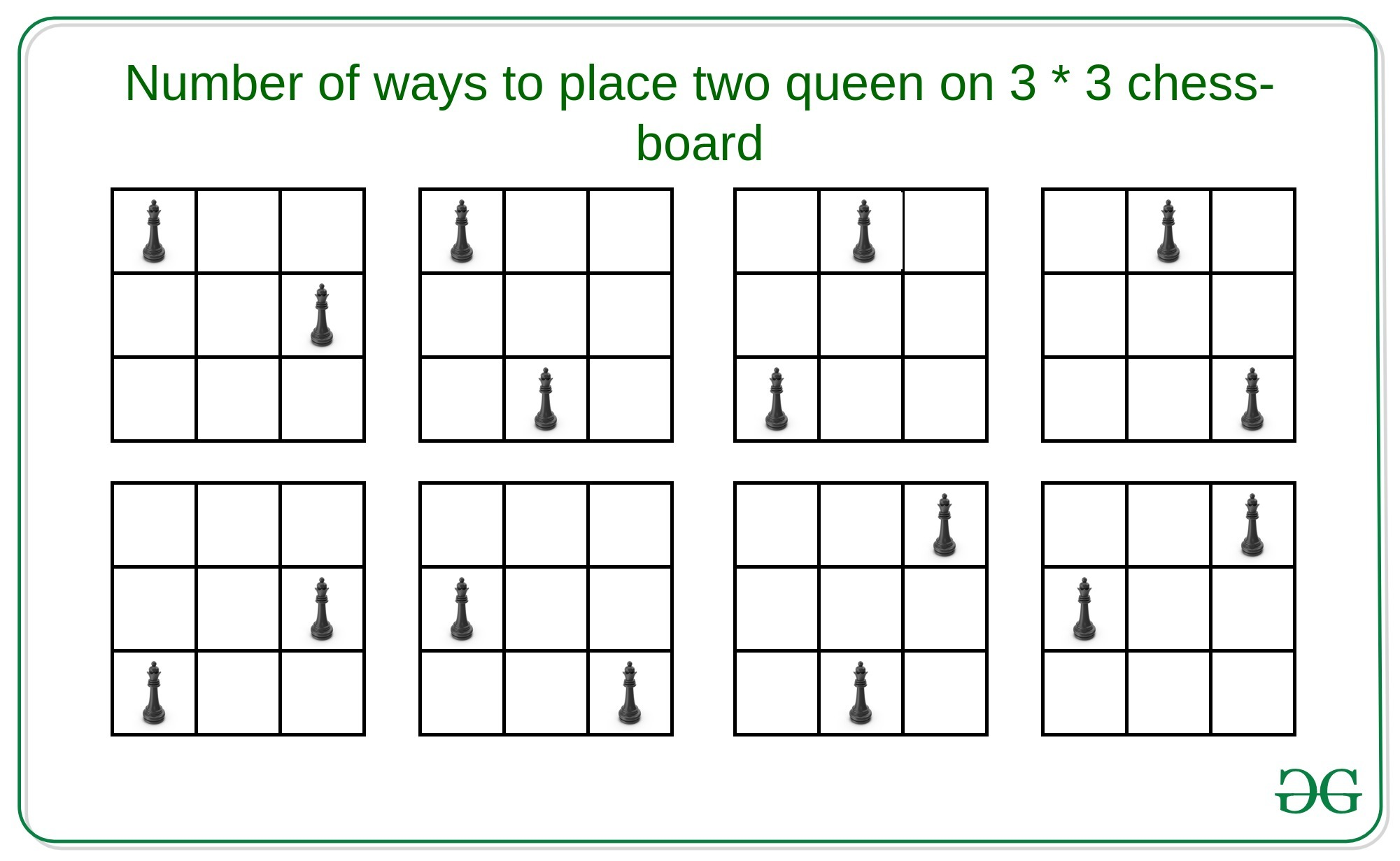# Number of ways to place two queens on a N*N chess-board

Given an integer N denoting a N * N chess-board, the task is to count the number of ways to place two queens on the board such that, they do no attack each other.

Examples:

Input: N = 9
Output: 2184
Explanation:
There are 2184 ways to place two queens on 9 * 9 chess-board.

Input: N = 3
Output: 8
Explanation:
There are 8 ways to place two queens on 3 * 3 chess-board.

## Recommended: Please try your approach on {IDE} first, before moving on to the solution.Naive Approach: A simple solution will be to choose two every possible position for the two queens on the N * N matrix and check that they are not in horizontal, vertical, positive diagonal or negative diagonal. If yes then increment the count by 1.
Time Complexity: O(N4)

Efficient Approach: The idea is to use combinations to compute the possible positions of the queens such that they do not attack each other. A useful observation is that it is quite easy to calculate the number of positions that a single queen attacks. That is –

```Number of positions a queen attack =
(N - 1) + (N - 1) + (D - 1)

Here,
// First N-1 denotes positions in horizontal direction
// Second N-1 denotes positions in vertical direction
// D = Number of positions in
positive and negative diagonal
```

If we do not place the queen on the last row and the last column then the answer will simply be the number of positions to place in a chessboard of (N-1)*(N-1), whereas if we place in the last column and last row then possible positions for queens will be 2*N – 1 and attacking at 3*(N – 1) positions. Therefore, the possible positions for the other queen will be N2 – 3*(N-1) – 1. Finally, there are (N-1)*(N-2) combinations where both queens are on the last row and last column. Therefore, the recurrence relation will be –

```Q(N) = Q(N-1) + (N2-3*(N-1)-1)-(N-1)*(N-2)

// By Induction
Q(N) = (N4)/2 - 5*(N3)/3 + 3*(N2)/2 - N/3
```

Below is the implementation of the above approach:

## C++

 `// C++ implementation to find the ` `// number of ways to place two  ` `// queens on the N * N chess board ` ` `  `#include ` ` `  `#define ll long long ` `using` `namespace` `std; ` ` `  `// Function to find number of valid  ` `// positions for two queens in the ` `// N * N chess board ` `ll possiblePositions(ll n) ` `{ ` `    ``ll term1 = ``pow``(n, 4); ` `    ``ll term2 = ``pow``(n, 3); ` `    ``ll term3 = ``pow``(n, 2); ` `    ``ll term4 = n / 3; ` `    ``ll ans = (``ceil``)(term1) / 2 -  ` `             ``(``ceil``)(5 * term2) / 3 +  ` `             ``(``ceil``)(3 * term3) / 2 - term4; ` `    ``return` `ans; ` `} ` ` `  `// Driver Code ` `int` `main() ` `{ ` `    ``ll n; ` `    ``n = 3; ` `     `  `    ``// Function Call ` `    ``ll ans = possiblePositions(n); ` `    ``cout << ans << endl; ` `    ``return` `0; ` `} `

## Java

 `// Java implementation to find the ` `// number of ways to place two  ` `// queens on the N * N chess board ` `class` `GFG{ ` ` `  `// Function to find number of valid  ` `// positions for two queens in the ` `// N * N chess board ` `static` `double` `possiblePositions(``double` `n) ` `{ ` `    ``double` `term1 = Math.pow(n, ``4``); ` `    ``double` `term2 = Math.pow(n, ``3``); ` `    ``double` `term3 = Math.pow(n, ``2``); ` `    ``double` `term4 = n / ``3``; ` `    ``double` `ans = (Math.ceil(term1 / ``2``)) -  ` `                 ``(Math.ceil(``5` `* term2) / ``3``) +  ` `                 ``(Math.ceil(``3` `* term3) / ``2``) - term4; ` ` `  `    ``return` `(``long``)ans; ` `} ` ` `  `// Driver Code ` `public` `static` `void` `main(String[] args) ` `{ ` `    ``double` `n; ` `    ``n = ``3``; ` `     `  `    ``// Function Call ` `    ``double` `ans = possiblePositions(n); ` `    ``System.out.print(ans + ``"\n"``); ` `} ` `} ` ` `  `// This code is contributed by sapnasingh4991 `

## C#

 `// C# implementation to find the ` `// number of ways to place two  ` `// queens on the N * N chess board ` `using` `System; ` ` `  `class` `GFG{ ` ` `  `// Function to find number of valid  ` `// positions for two queens in the ` `// N * N chess board ` `static` `double` `possiblePositions(``double` `n) ` `{ ` `    ``double` `term1 = Math.Pow(n, 4); ` `    ``double` `term2 = Math.Pow(n, 3); ` `    ``double` `term3 = Math.Pow(n, 2); ` `    ``double` `term4 = n / 3; ` `    ``double` `ans = (Math.Ceiling(term1 / 2)) -  ` `                 ``(Math.Ceiling(5 * term2) / 3) +  ` `                 ``(Math.Ceiling(3 * term3) / 2) - term4; ` ` `  `    ``return` `(``long``)ans; ` `} ` ` `  `// Driver Code ` `public` `static` `void` `Main(String[] args) ` `{ ` `    ``double` `n; ` `    ``n = 3; ` `     `  `    ``// Function Call ` `    ``double` `ans = possiblePositions(n); ` `    ``Console.Write(ans + ``"\n"``); ` `} ` `} ` ` `  `// This code is contributed by Amit Katiyar `

Output:

```8
```

Time Complexity: O(1)

Don’t stop now and take your learning to the next level. Learn all the important concepts of Data Structures and Algorithms with the help of the most trusted course: DSA Self Paced. Become industry ready at a student-friendly price.

My Personal Notes arrow_drop_upCheck out this Author's contributed articles.

If you like GeeksforGeeks and would like to contribute, you can also write an article using contribute.geeksforgeeks.org or mail your article to contribute@geeksforgeeks.org. See your article appearing on the GeeksforGeeks main page and help other Geeks.

Please Improve this article if you find anything incorrect by clicking on the "Improve Article" button below.